25 Third Grade Math Games and Activities That Really Multiply The Fun
Home » Uncategorized  »  25 Third Grade Math Games and Activities That Really Multiply The Fun
25 Third Grade Math Games and Activities That Really Multiply The Fun

When you get to third grade, math takes a pretty big leap forward. Common Core standards include multiplication and division, basic geometry, and rounding, among others. These third grade math games and activities will help your students master these key skills in fun and engaging ways.

## 1. Count your dots to learn multiplication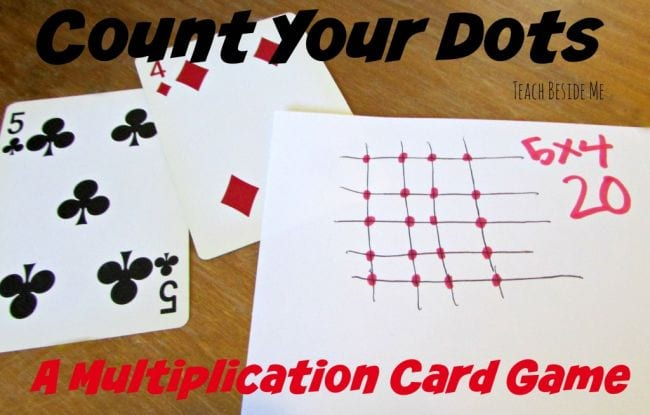Multiplication is a new skill for third grade math students, but it builds on concepts they’ve mastered in earlier grades. This card game helps them make the connections. Each player flips two cards, then draws a grid and makes dots where the lines join. They count the dots, and the person with the most keeps all the cards.

Learn more: Teach Beside Me

## 2. Punch holes for multiplication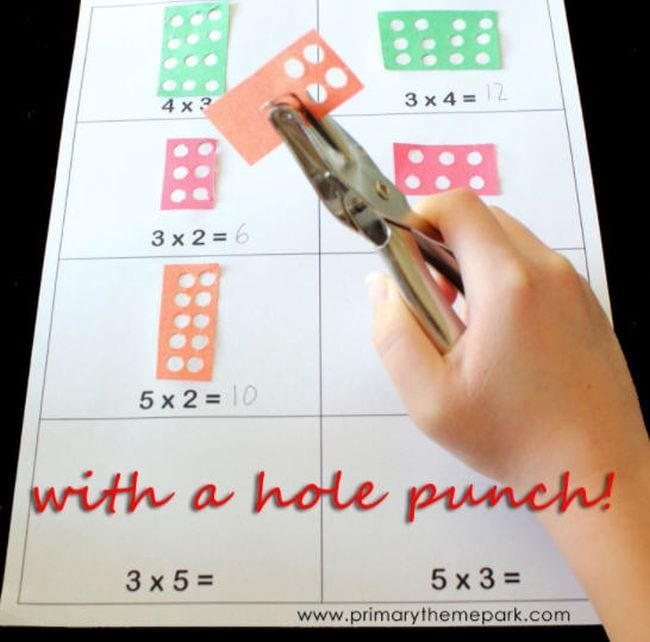Arrays are a popular way to teach multiplication skills, and this is a fun activity that uses the concept. Pull out some scrap paper and cut out squares or rectangles. Then use a hole punch to make dot arrays to represent multiplication equations.

Learn more: Primary Theme Park

## 3. Flip dominoes and multiply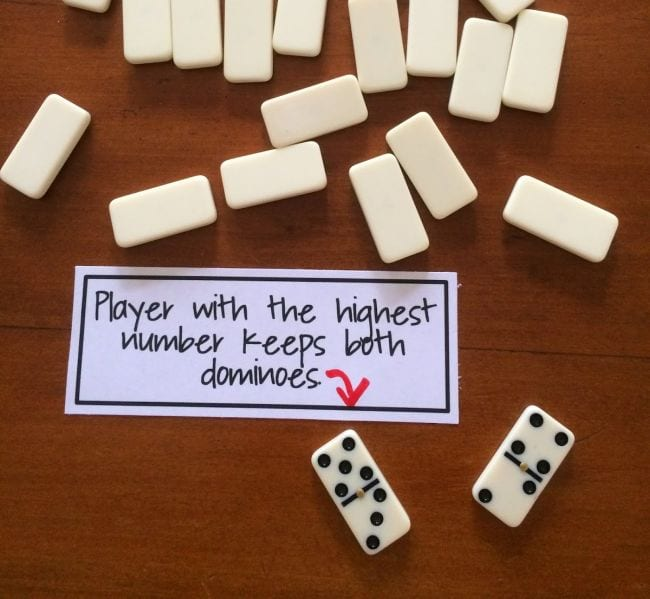Eventually, kids will have to memorize multiplication facts, and this quick and easy domino game can help. Each player flips a domino and multiplies the two numbers. The one with the highest product gets both dominoes.

Learn more: Fun Games 4 Learning

## 4. Search for the multiplication equations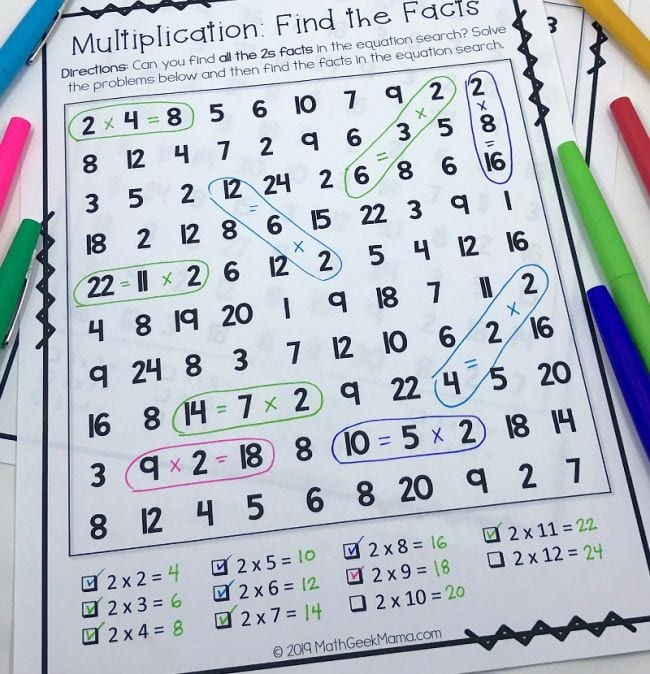It’s like a word search, but for multiplication facts! Grab the free printables at the link.

Learn more: Math Geek Mama

## 5. Repurpose a Guess Who? board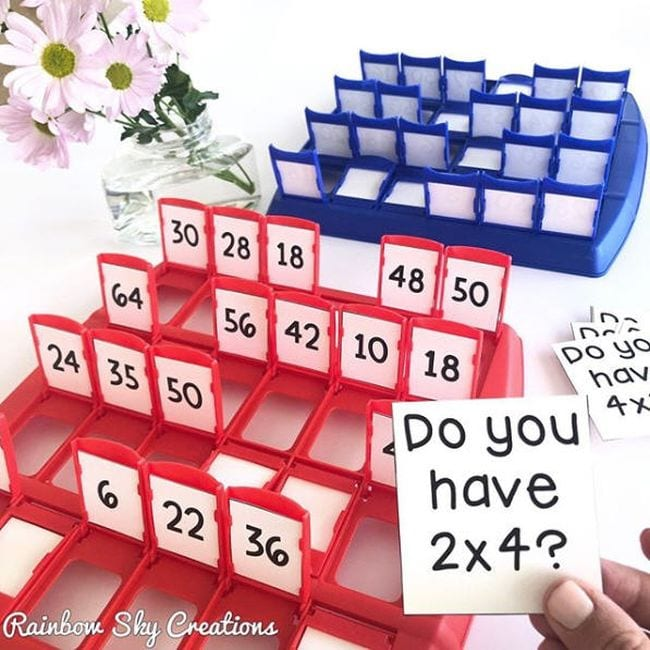One more multiplication game, using a Guess Who? game board. (You could also do this with division facts.)

Learn more: Rainbow Sky Creations/Instagram

## 6. Craft division fact flowers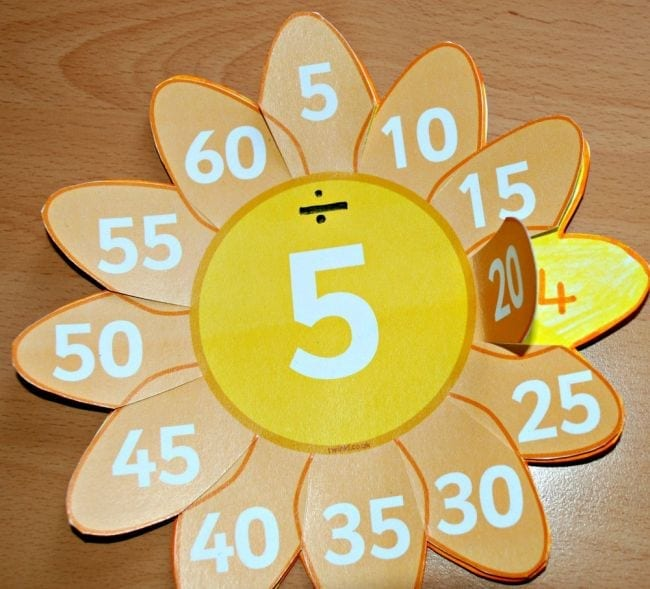This is so much more fun than flashcards! Make flowers for each number and use them to practice division facts.

Learn more: Ofamily Learning Together

## 7. Roll and race to practice division factsMultiplication and division go hand-in-hand in third grade math. This free printable game has kids rolling the die, trying to be the first to correctly answer all the problems in one row. Get the printable at the link.

Learn more: Jennifer Findley

## 8. Divide and conquer division pairs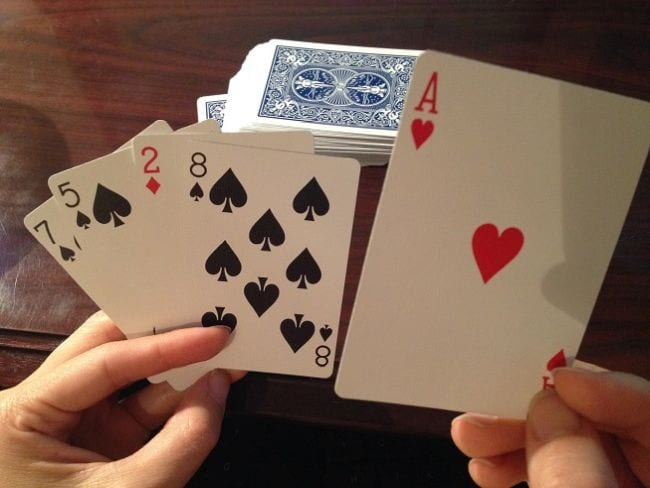Think Go Fish, but instead of matching pairs, the aim is to match two cards in which one can divide evenly into the other. For instance, 8 and 2 are a pair since 8 ÷ 2 = 4.

Learn more: cuppacocoa

## 9. Figure out the missing sign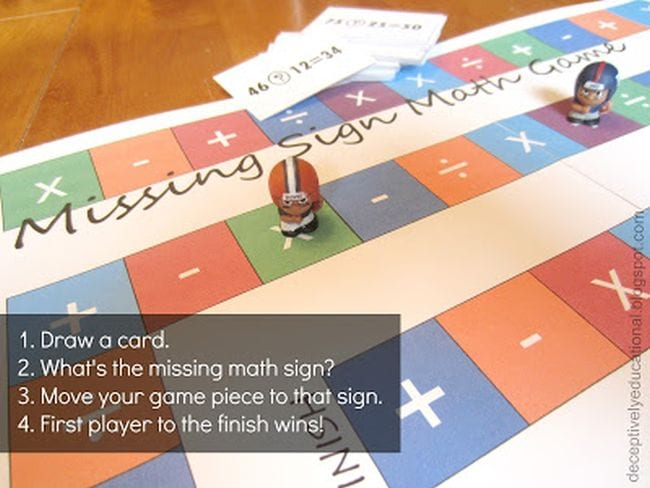Once kids know all four types of arithmetic, they should be able to work backward to see which sign is missing in an equation. The free printable board game at the link challenges them to do just that.

Learn more: Deceptively Educational

## 10. Use sticky notes to play Can You Make It?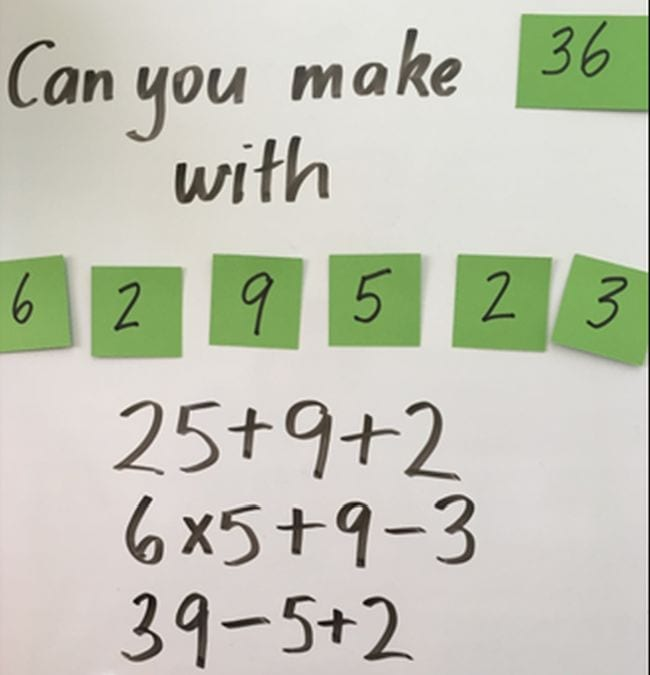Give students a series of numbers on sticky notes along with a target number. Then see if they can make an equation (or multiple equations) that meet the target.

Learn more: Games for Learning

## 11. Introduce rounding with a card game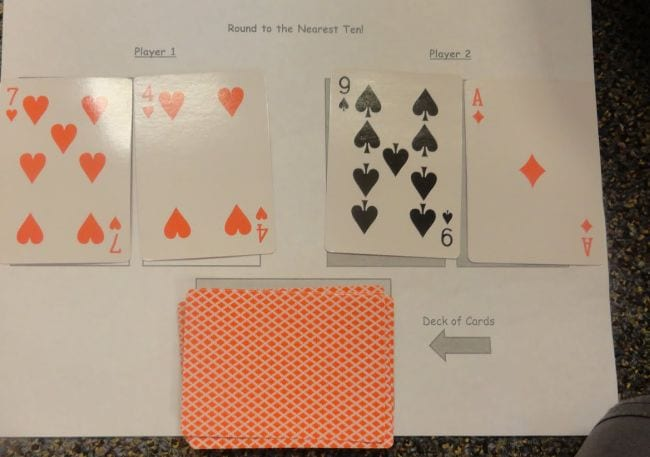Third grade math students learn about rounding numbers. This card game has them facing off to flip two cards each and round the resulting number to the nearest 10. The one whose number is largest keeps all the cards.

Learn more: Adventures in Third Grade

## 12. Roll it and round it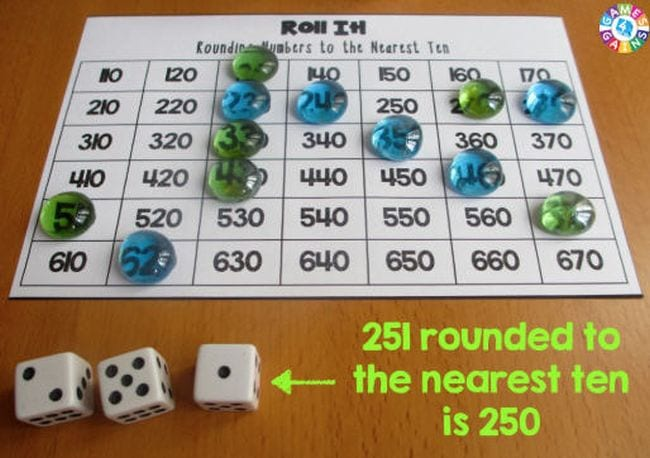Use this free printable board to play Roll It! for more rounding practice. Students roll three dice, then arrange them into a number. They round to the nearest 10 and mark it off on their board. The goal is to be the first to complete a row.

Learn more: Games 4 Gains

## 13. Use LEGO bricks to learn fractions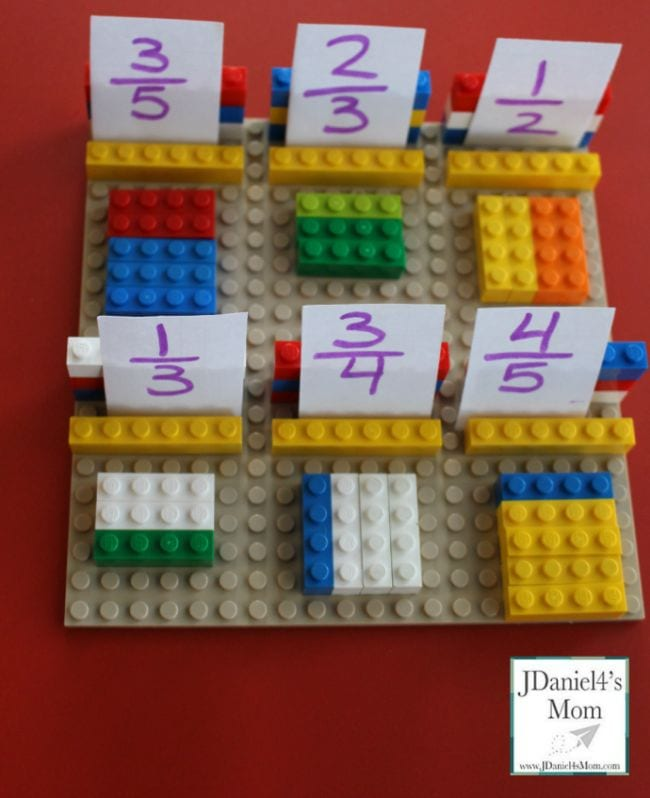In third grade math, students start learning fractions in earnest. Playing with LEGOs makes it fun! Kids draw cards and use colored bricks to represent the fraction shown. Check out even more ways to use LEGO bricks for math.

Learn more: JDaniel4’s Mom

## 14. Match up plastic eggs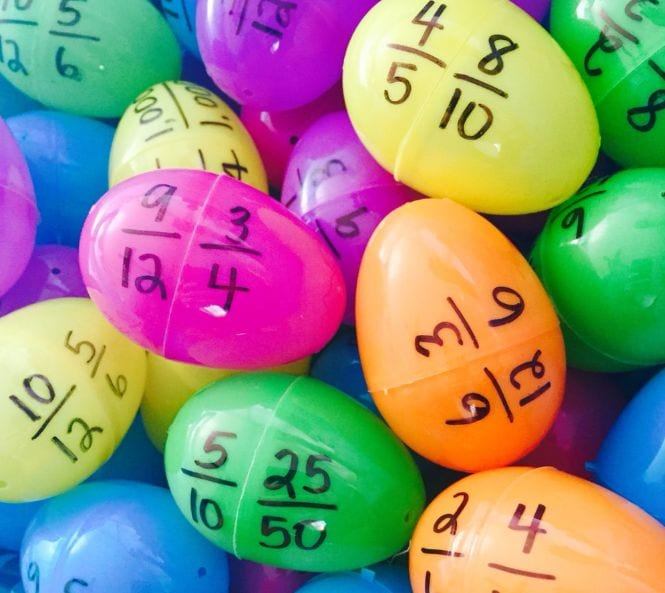Try a different kind of egg hunt to practice equivalent fractions. Write fractions on each half, then have kids find them and make the proper matches. (Make this harder by mixing up the colors!) Check out our other ways to use plastic eggs in the classroom.

## 15. Play fraction match-up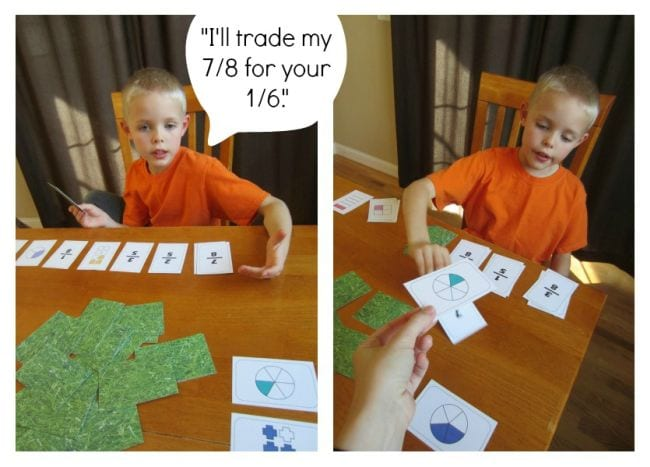Grab the free printable cards at the link and work to make matches between the pictures and the fractions they represent.

Learn more: Deceptively Educational

## 16. Declare a fraction war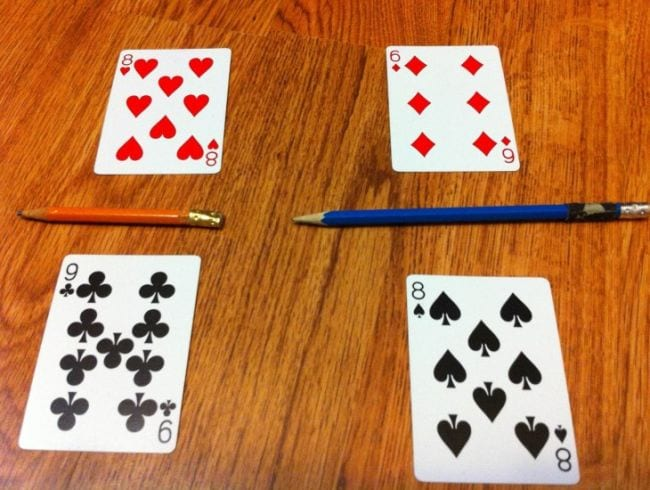Each player flips two cards and lays them out as a fraction. They decide which fraction is greatest, with the winner keeping all the cards. Comparing fractions gets a little tricky, but if kids plot them on a fraction number line first, they’ll be practicing two skills at once.

Learn more: Math File Folder Games

## 17. Master telling time to the minute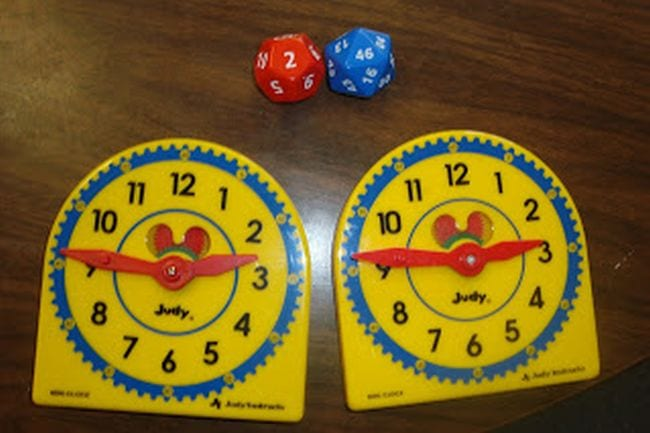You’ll need some polyhedral dice for this third grade math game. Kids roll the dice and race to be the first to represent the proper time on their clock.

Learn more: The Elementary Math Maniac

## 18. Match up LEGO bricks to tell time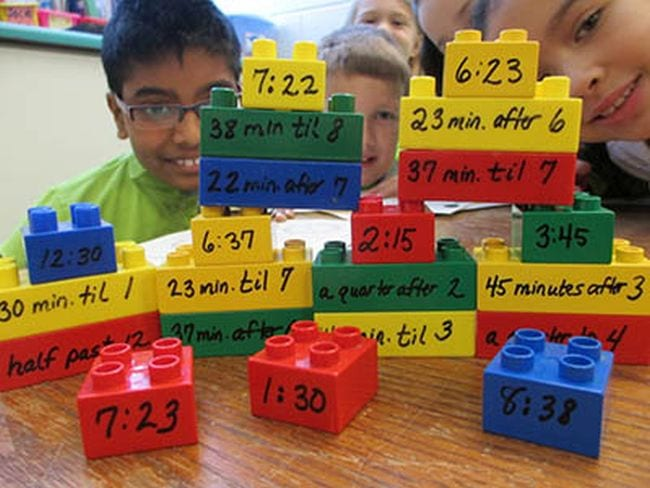Write times in a variety of ways on LEGO bricks. Then have students see how quickly they can match them up.

Learn more: Scholastic

## 19. Explore perimeter and area with Array Capture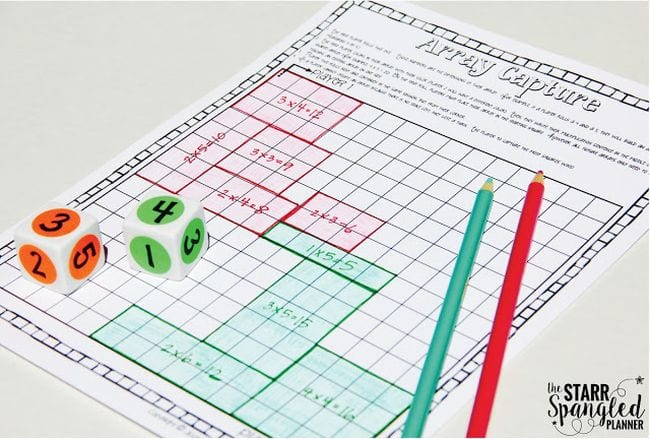Geometry takes on more importance in third grade math, as students learn area and perimeter. This fun and simple game covers both, and all you need to play is graph paper and some dice.

Learn more: Teaching With Jillian Starr

## 20. Draw perimeter people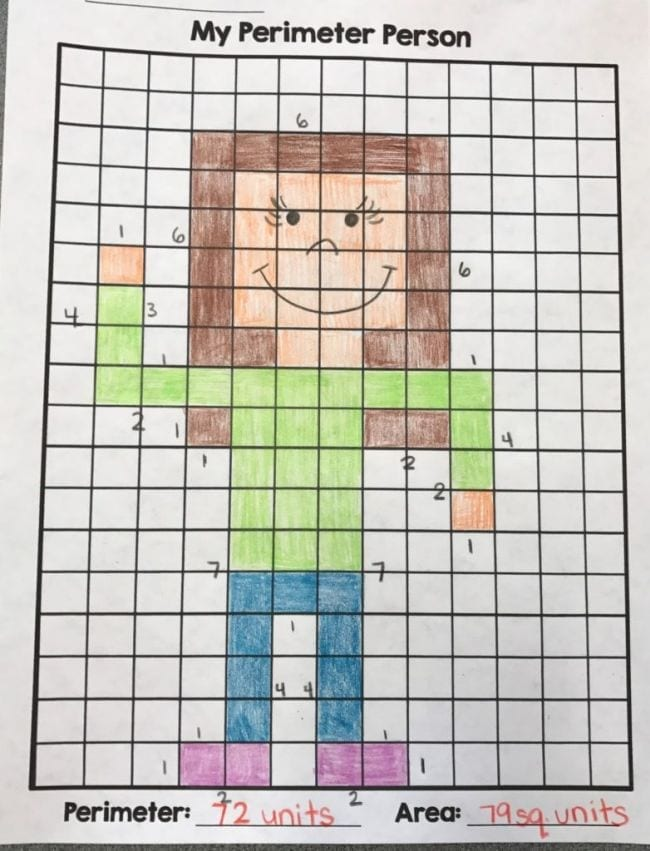Have kids draw self-portraits on graph paper, then calculate the perimeter and area of their block people. Cute and fun!

Learn more: A Word From Third

## 21. Build LEGO puzzles for more area and perimeter practice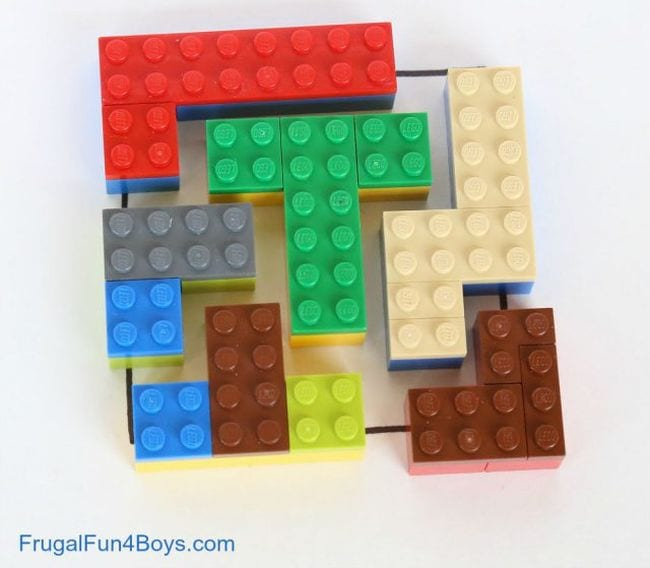The challenge: Build a 10×10 puzzle from LEGO bricks for your friends to solve. Have kids figure the perimeter and area of each puzzle piece too.

Learn more: Frugal Fun for Boys and Girls

## 22. Color a polygon quilt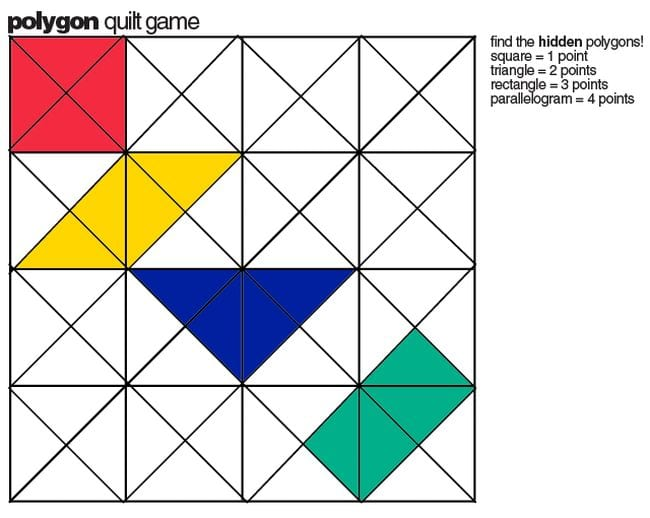Players take turns coloring in four connected triangles at a time, earning points for the shape they create. It’s a fun way to practice polygons.

Learn more: E is for Explore

## 23. Play quadrilateral bingo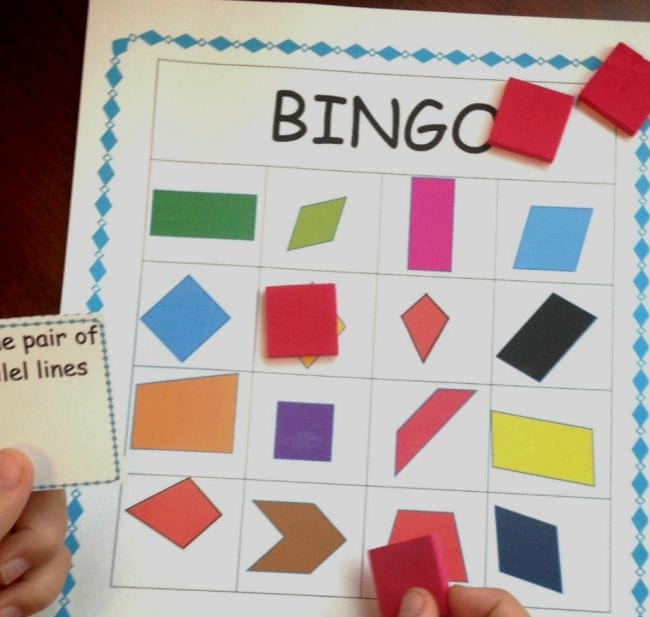Every square is a rectangle, but not all rectangles are squares. Get a handle on quirky quadrilaterals with this free printable bingo game.

Learn more: You’ve Got This Math

## 24. Roll and add to build bar graphs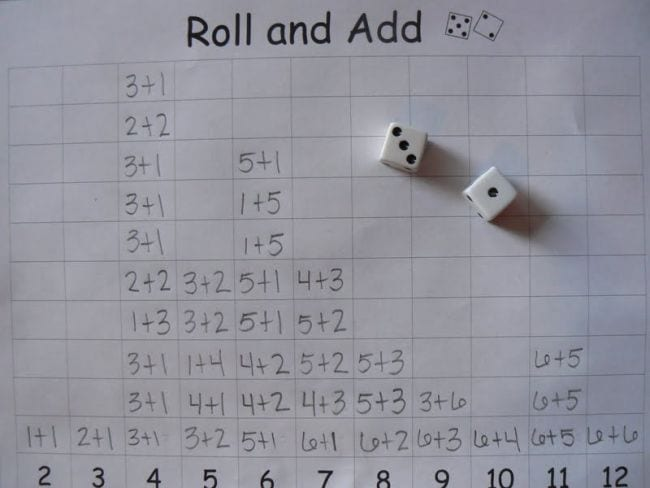First, students roll dice and add the two numbers, writing the equation in the correct sum column. Repeat as many times as you like. Then, ask questions to analyze the data. Which sum did they roll most often? How many more times did they roll the highest than the lowest? It’s an interesting way to review addition facts and work on graphing.

Learn more: Mrs T’s First Grade Class

## 25. Solve math riddles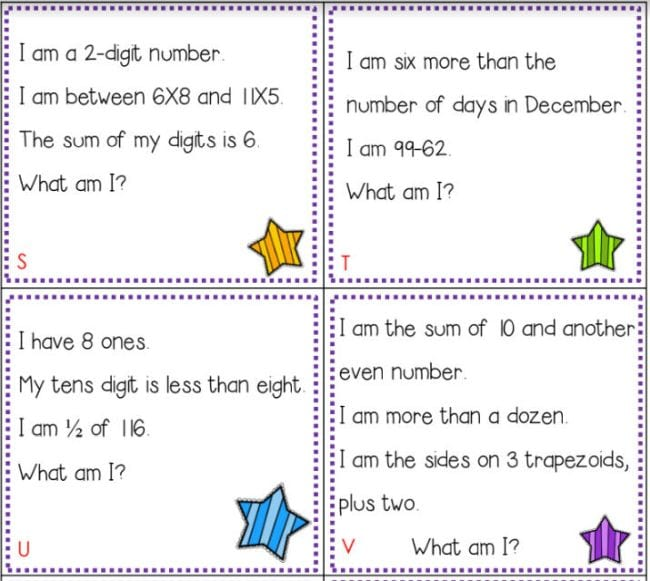Put together all your third grade math skills to solve these math riddles. Get a free printable set at the link.

Learn more: Primary Inspiration

### Plus, get all the latest teaching tips and tricks, straight to your inbox, when you sign up for our newsletters!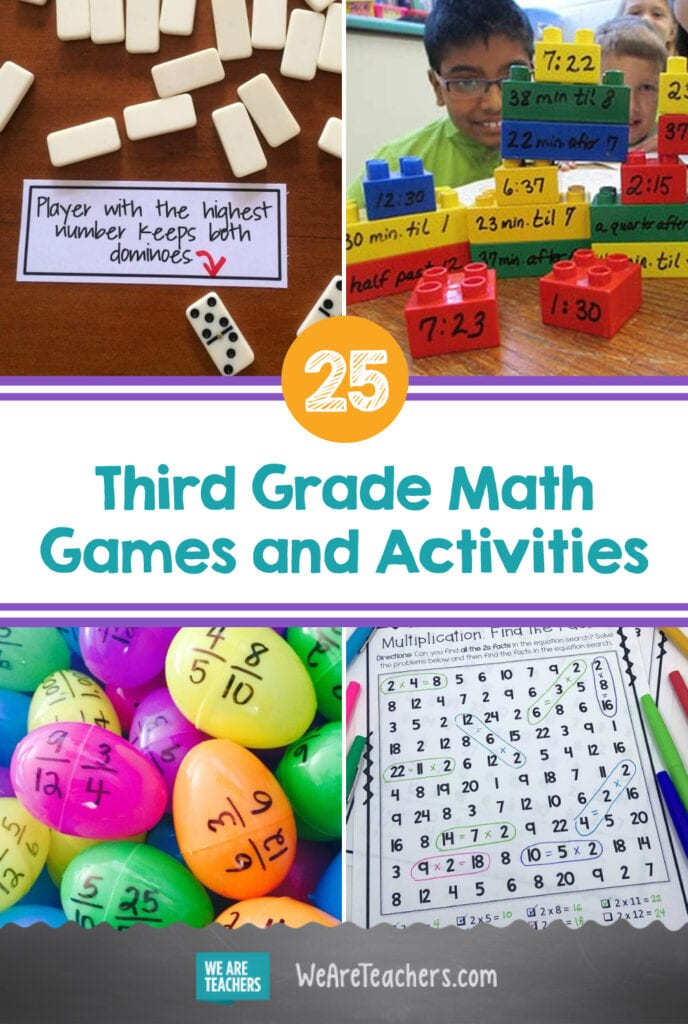Source link